Examples from Takashi Kojima's book "Advances Abacus, Theory and Practice"
(All examples are taken from the chapter "Other Methods of Multiplication.")

The Elimination of the Initial Digit of a Multiplier Beginning with One

Multiplication can also be simplified by leaving out the initial digit on the multiplier. The Japanese technical term for this may be translated as the initial-digit-one-elimination-multiplication.

Example 1.  75 x 103 = 7,725

Fig. 1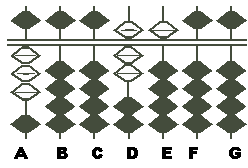Step 1: Set the multiplicand 75 on DE and the 3 of the multiplier 103 on A. (Fig. 1)

Fig. 2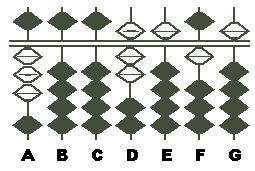Step 2: Multiply 3 on A by 5 on E, set the product 15 on FG. This makes a total of 7,515 on DEFG.  (Fig. 2)

Fig. 3Step 3: Next multiply the 3 on A by the 7 on D, add the product 21 to 51 on EF. This gives you a total of 7725 on EFGH, which is the answer. (Fig.3)

Example 2.  78 x 13 = 1,014

Fig. 1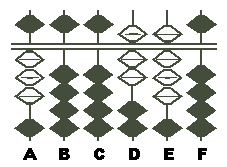Step 1: Set the multiplicand 78 on DE and the 3 of the multiplier 13 on A. (Fig.1)

Fig. 2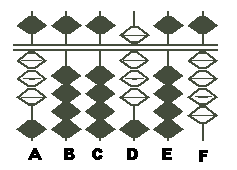Step 2: Multiplying the 3 on A by the 8 on E, set the product 24 on EF. This makes a total of 804 on DEF. (Fig.2)

(Note: However, you must remember till the next step that the digit which was originally on D was not 8 but 7.)

Fig. 3Step 3: Multiplying the 3 on A by the 7, which you remember was on D, set the product 21 on DE. This gives you, on CDEF, a total of 1,014, which is the answer. (Fig.3)

Example 3.  An article was bought for \$250 and sold at a gain of 6.8%. Find the selling price. Since the selling price is determined by adding the cost and the profit, it can be found by the following multiplication.

\$250 X (1 + 0.068) = \$250 X 1.068 = \$267

There are two methods for working this multiplication. One is the standard method of multiplication and the other the method of eliminating the initial digit one of the multiplier. The latter operation is shown below.

Fig. 1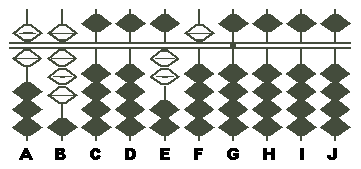Step 1: Set the multiplicand 250 on EFG, with G as the unit rod, and set the 68 of the multiplier on AB. (Fig.1)

(Note: When the multiplier is a mixed decimal, it is generally advisable to set its unit figure on a unit rod. But experts usually do not bother to, since the digit 1 is not used in calculation. As long as you remember that 68 stands for 1.068, it matters little to set the unit digit of the multiplier on the unit rod.)

Fig. 2Step 2: Since the multiplier is a four-digit number, suppose that 250 has been multiplied by 1000, producing 250,000 on EFGHIJ, with G as the unit rod.  Now multiplying the 6 on A by the 5 on F, set 30 on GH. (Fig.2)

(Note: As both the 6 on A and the 5 on F are in the tens place, the product 30 must be set on GH.)

Fig. 3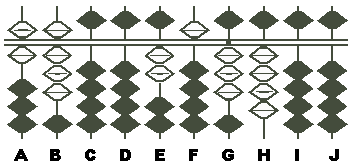Step 3: Next multiplying the 8 on B by same 5 on F, set the product 40 on HI. This gives you 34 on GH. (Fig.3)

Fig. 4Step 4: Next multiplying the 6 on A by the 2 on E, ass the product 12 to the 53 on FG. This gives you 654 on FGH. (Fig.4)

Fig. 5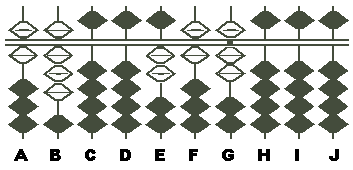Step 5: Finally multiplying the 8 on B by the same 2 on E, add the product 16 to the 54 on GH. This gives you, on EFG, a total of 267, which is the answer. (Fig.5)

- As written by Takashi Kojima
from his book, "Advances Abacus, Theory and Practice"
Publisher: Charles E. Tuttle, 1954